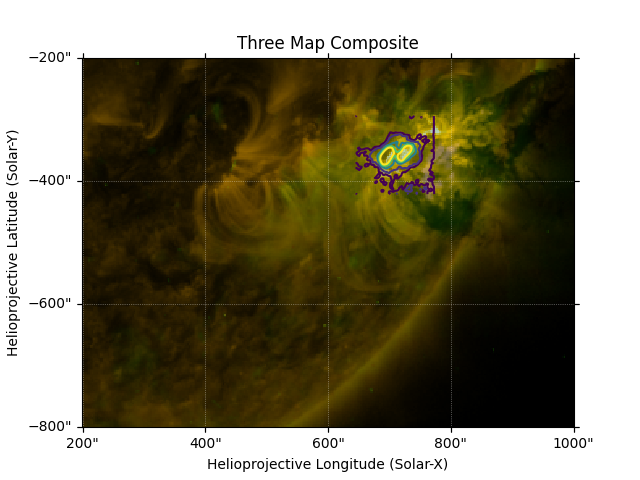# Creating a Composite Plot with Three Maps#

In this example, a composite plot is created with three maps. It demonstrates how to specify contour levels, transparency, and ordering when overlaying multiple maps.

import matplotlib.pyplot as plt

import astropy.units as u
from astropy.coordinates import SkyCoord

import sunpy.data.sample
from sunpy.coordinates import Helioprojective
from sunpy.map import Map


First, we will import sample data from EIT, RHESSI, and AIA. The EIT data shows a hot region of the solar corona, while AIA shows the cooler upper region of the corona. RHESSI data is focused on a solar flare, and will be plotted using contours.

eit = Map(sunpy.data.sample.EIT_195_IMAGE)
rhessi = Map(sunpy.data.sample.RHESSI_IMAGE)
aia = Map(sunpy.data.sample.AIA_171_IMAGE)


Before we plot the image, let’s reproject the off-disk AIA coordinates onto a spherical screen at the same distance as the EIT map, so they can be overlaid. Next, zoom in around the solar flare so the RHESSI contours are visible. Also, specify the RHESSI contour levels to be plotted.

with Helioprojective.assume_spherical_screen(eit.observer_coordinate):
aia = aia.reproject_to(eit.wcs)

bottom_left = [200, -800] * u.arcsec
top_right = [1000, -200] * u.arcsec
eit_smap = eit.submap(SkyCoord(*bottom_left, frame=eit.coordinate_frame),
top_right=SkyCoord(*top_right, frame=eit.coordinate_frame))
aia_smap = aia.submap(SkyCoord(*bottom_left, frame=aia.coordinate_frame),
top_right=SkyCoord(*top_right, frame=aia.coordinate_frame))
levels = [5, 10, 20, 30, 40]*u.percent


When creating the plot, choose which Map will be used to create the WCS Axes that the other Maps will be plotted with. The EIT map is plotted first, followed by the AIA map, and lastly the RHESSI contours. Transparency is changed to 70% on the AIA map by specifying the parameter alpha, and the image data is autoaligned to the EIT WCS Axes. The parameter zorder specifies how each plot is layered (0 is plotted first and 1 is layered on top of 0, and so on).

fig = plt.figure()
eit_smap.plot(axes=ax, clip_interval=(1, 99.9)*u.percent, zorder=0)
aia_smap.plot(axes=ax, clip_interval=(1, 99.97)*u.percent, alpha=0.7,
autoalign=True, zorder=1)
rhessi.draw_contours(axes=ax, levels=levels, zorder=2)
ax.set_title("Three Map Composite")

plt.show()INFO: Missing metadata for solar radius: assuming the standard radius of the photosphere. [sunpy.map.mapbase]